## 递归模型的应用场景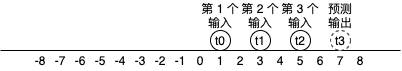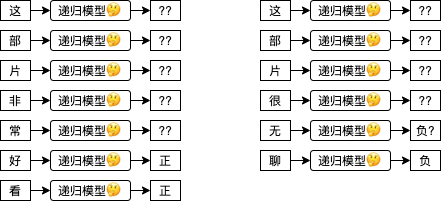model = MyRecursiveModel()
model('这')
model('部')
model('片')
model('非')
model('常')
model('好')
last_output = model('看')
print(last_output)


## 最简单的递归模型 - RNN (tanh)

RNN tanh (Recurrent Neural Network - tanh) 是最简单的递归模型，计算公式如下，数学不好的第一印象可能会觉得妈呀一看数学公式就头昏脑胀了🙀，我们先一个个参数来分析，H 是递归模型内部记录的一个隐藏值矩阵，Ht 代表当前时序的值，而 H(t-1) 代表前一个时序的值，t 可以置换到具体的数字，例如 Ht0 代表隐藏值矩阵最开始的状态 (一般会使用 0 初始化)，Ht1 代表隐藏值矩阵第一次更新以后的状态，Ht2 代笔隐藏值矩阵第二次更新以后的状态，计算 Ht1H(t-1) 代表 Ht0，计算 Ht2H(t-1) 代表 Ht1Wx 是针对输入的权重值，Bx 是针对输入的偏移值，Wh 是针对隐藏的权重值，Bh 是针对隐藏的偏移值；tanh 用于把实数转换为 -1 ~ 1 之间的范围。这个公式和之前我们看到的人工神经元很相似，只是把每一次的输入和当前隐藏值经过计算后的值加在一起，然后使用 tanh 作为激活函数生成新的隐藏值，隐藏值会当作每一次的输出使用。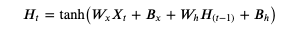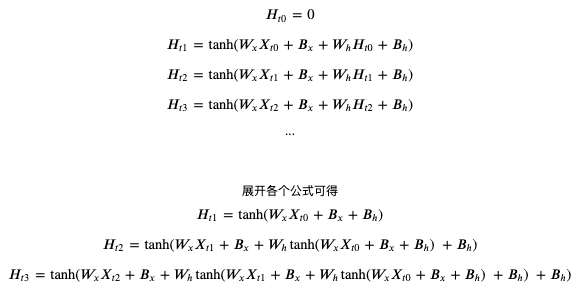(看不清请在新标签单独打开图片，或者另存为到本地以后查看)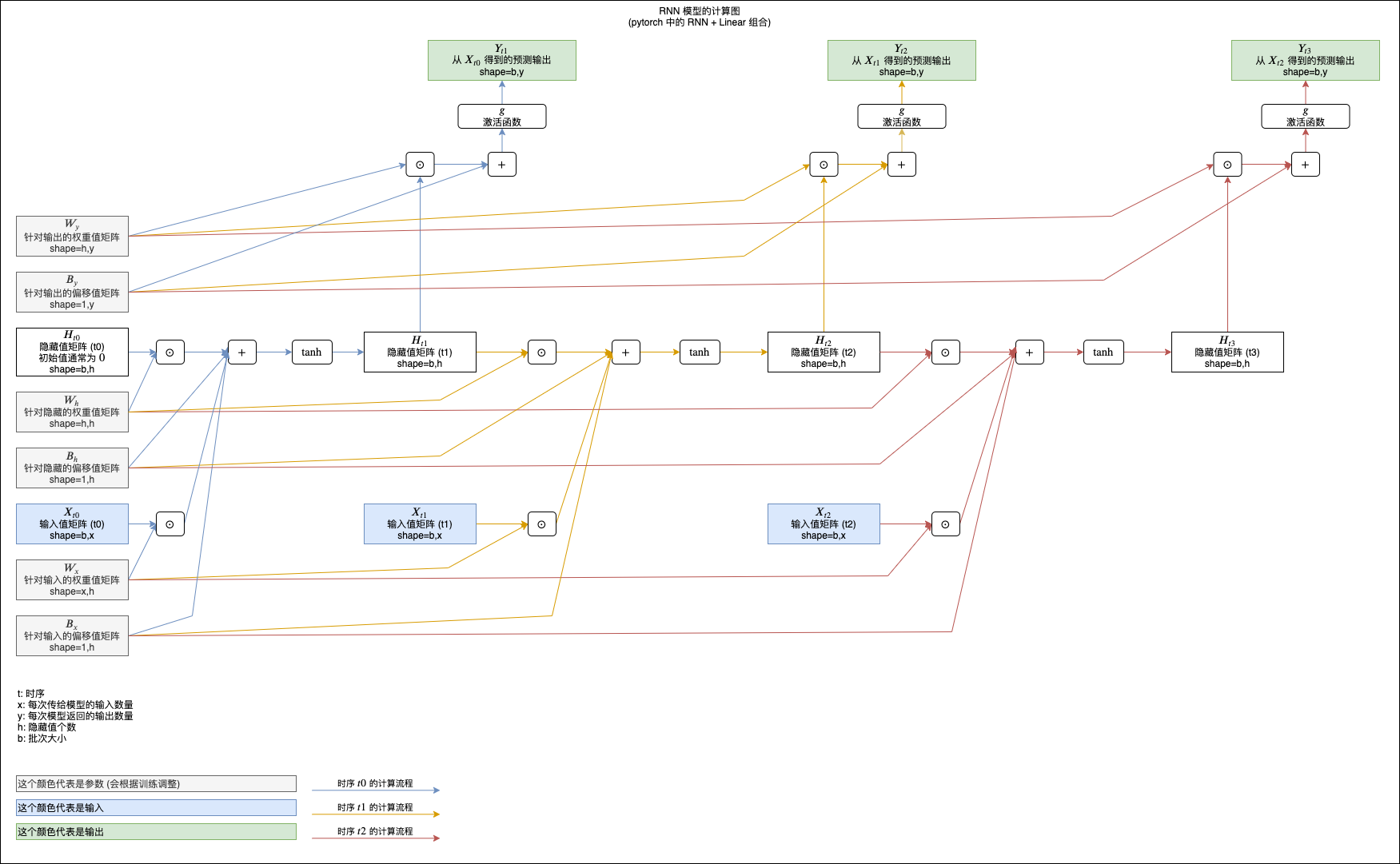## 在 pytorch 中使用 RNN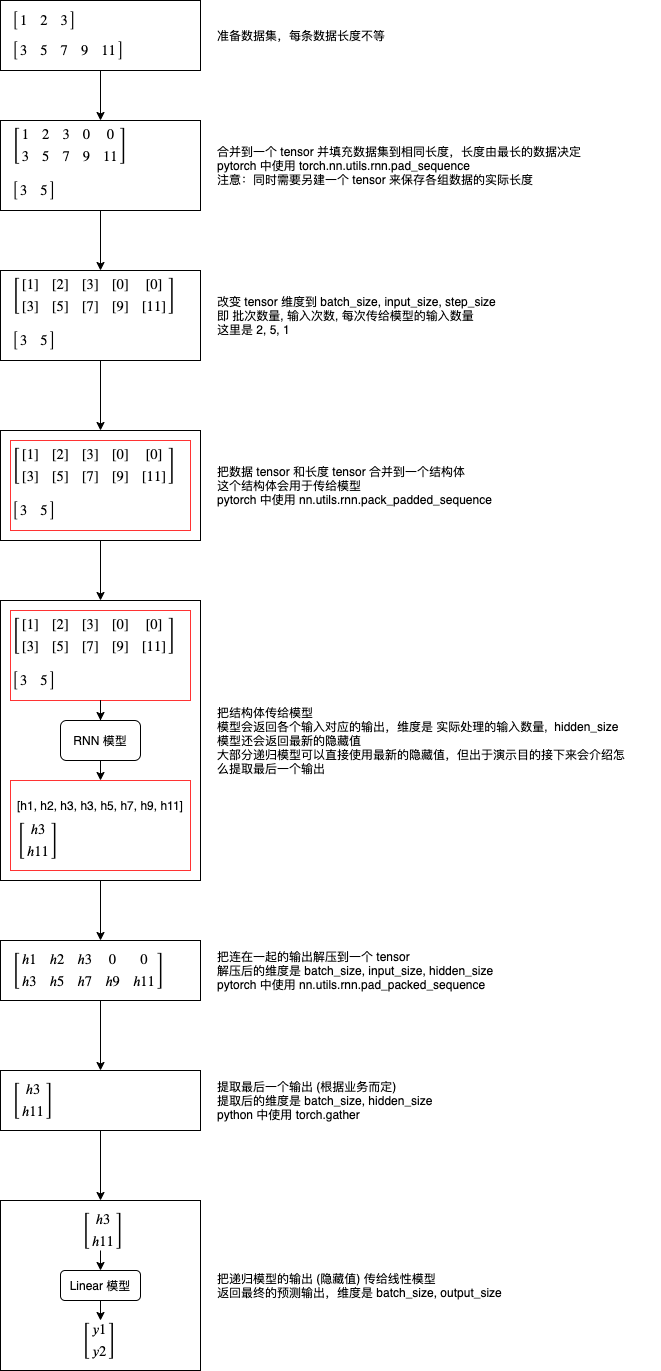# 引用 pytorch 类库
>>> import torch

# 准备数据集
>>> data1 = torch.tensor([1, 2, 3], dtype=torch.float)
>>> data2 = torch.tensor([3, 5, 7, 9, 11], dtype=torch.float)
>>> datalist = [data1, data2]

# 合并到一个 tensor 并填充数据集到相同长度
# 这里使用 batch_first 指定第一维度为批次数量
tensor([[ 1.,  2.,  3.,  0.,  0.],
[ 3.,  5.,  7.,  9., 11.]])

# 另建一个 tensor 来保存各组数据的实际长度
>>> lengths = torch.tensor([len(x) for x in datalist])
>>> lengths
tensor([3, 5])

# 建立 RNN 模型，每次接收 1 个输入，内部拥有 8 个隐藏值 (每次都会返回 8 个最新的隐藏值)
# 指定 num_layers 可以在内部叠加 RNN 模型，这里不叠加所以只指定 1
>>> rnn_model = torch.nn.RNN(input_size = 1, hidden_size = 8, num_layers = 1, batch_first = True)

# 建立 Linear 模型，每次接收 8 个输入 (RNN 模型返回的隐藏值)，返回 1 个输出
>>> linear_model = torch.nn.Linear(in_features = 8, out_features = 1)

# 改变 tensor 维度到 batch_size, input_size, step_size
# 即 批次数量, 输入次数, 每次传给模型的输入数量
>>> x
tensor([[[ 1.],
[ 2.],
[ 3.],
[ 0.],
[ 0.]],

[[ 3.],
[ 5.],
[ 7.],
[ 9.],
[11.]]])

# 把数据 tensor 和长度 tensor 合并到一个结构体
# 这样做的意义是可以避免 RNN 计算填充的那些 0
# enforce_sorted 表示数据事先没有排序过，如果不指定这个选项会出错
>>> packed = torch.nn.utils.rnn.pack_padded_sequence(x, lengths, batch_first=True, enforce_sorted=False)
>>> packed
PackedSequence(data=tensor([[ 3.],
[ 1.],
[ 5.],
[ 2.],
[ 7.],
[ 3.],
[ 9.],
[11.]]), batch_sizes=tensor([2, 2, 2, 1, 1]), sorted_indices=tensor([1, 0]), unsorted_indices=tensor([1, 0]))

# 把结构体传给模型
# 模型会返回各个输入对应的输出，维度是 实际处理的输入数量,  hidden_size
# 模型还会返回最新的隐藏值
>>> output, hidden = rnn_model(packed)
>>> output
PackedSequence(data=tensor([[-0.3055,  0.2916,  0.2736, -0.0502, -0.4033, -0.1438, -0.6981,  0.6284],
[-0.2343,  0.2279,  0.0595,  0.1867, -0.2527, -0.0518, -0.1913,  0.5276],
[-0.0556,  0.2870,  0.3035, -0.3519, -0.4015,  0.1584, -0.9157,  0.6472],
[-0.1488,  0.2706,  0.1115, -0.0131, -0.2350,  0.1252, -0.4981,  0.5706],
[-0.0179,  0.1201,  0.4751, -0.5256, -0.3701,  0.1289, -0.9834,  0.7087],
[-0.1094,  0.1283,  0.1698, -0.1136, -0.1999,  0.1847, -0.7394,  0.5756],
[ 0.0426,  0.1866,  0.5581, -0.6716, -0.4857,  0.0039, -0.9964,  0.7603],
[ 0.0931,  0.2418,  0.6602, -0.7674, -0.6003, -0.0989, -0.9991,  0.8172]],
grad_fn=<CatBackward>), batch_sizes=tensor([2, 2, 2, 1, 1]), sorted_indices=tensor([1, 0]), unsorted_indices=tensor([1, 0]))
>>> hidden
tensor([[[-0.1094,  0.1283,  0.1698, -0.1136, -0.1999,  0.1847, -0.7394,
0.5756],
[ 0.0931,  0.2418,  0.6602, -0.7674, -0.6003, -0.0989, -0.9991,

# 把连在一起的输出解压到一个 tensor
# 解压后的维度是 batch_size, input_size, hidden_size
# 注意第二个返回值是各组输出的实际长度，等于之前的 lengths，所以我们不需要
>>> unpacked, _ = torch.nn.utils.rnn.pad_packed_sequence(output, batch_first=True)
>>> unpacked
tensor([[[-0.2343,  0.2279,  0.0595,  0.1867, -0.2527, -0.0518, -0.1913,
0.5276],
[-0.1488,  0.2706,  0.1115, -0.0131, -0.2350,  0.1252, -0.4981,
0.5706],
[-0.1094,  0.1283,  0.1698, -0.1136, -0.1999,  0.1847, -0.7394,
0.5756],
[ 0.0000,  0.0000,  0.0000,  0.0000,  0.0000,  0.0000,  0.0000,
0.0000],
[ 0.0000,  0.0000,  0.0000,  0.0000,  0.0000,  0.0000,  0.0000,
0.0000]],

[[-0.3055,  0.2916,  0.2736, -0.0502, -0.4033, -0.1438, -0.6981,
0.6284],
[-0.0556,  0.2870,  0.3035, -0.3519, -0.4015,  0.1584, -0.9157,
0.6472],
[-0.0179,  0.1201,  0.4751, -0.5256, -0.3701,  0.1289, -0.9834,
0.7087],
[ 0.0426,  0.1866,  0.5581, -0.6716, -0.4857,  0.0039, -0.9964,
0.7603],
[ 0.0931,  0.2418,  0.6602, -0.7674, -0.6003, -0.0989, -0.9991,

# 提取最后一个输出 (根据业务而定)
# 提取后的维度是 batch_size, hidden_size
# 可以看到使用 RNN tanh 时最后一个输出的值等于之前返回的 hidden
>>> last_hidden = unpacked.gather(1, (lengths - 1).reshape(-1, 1, 1).repeat(1, 1, unpacked.shape))
>>> last_hidden
tensor([[[-0.1094,  0.1283,  0.1698, -0.1136, -0.1999,  0.1847, -0.7394,
0.5756]],

[[ 0.0931,  0.2418,  0.6602, -0.7674, -0.6003, -0.0989, -0.9991,

# 把 RNN 模型返回的隐藏值传给 Linear 模型，得出预测输出
>>> predicted = linear_model(last_hidden)
>>> predicted
tensor([[[0.1553]],



## RNN 的简单例子

1,3,5,7,9


import os
import sys
import torch
import gzip
import itertools
from torch import nn
from matplotlib import pyplot

class MyModel(nn.Module):
"""根据球的历史位置预测将来位置的模型，假设球只能双方向匀速移动"""
def __init__(self):
super().__init__()
self.rnn = nn.RNN(
input_size = 1,
hidden_size = 8,
num_layers = 1,
batch_first = True
)
self.linear = nn.Linear(in_features=8, out_features=1)

def forward(self, x, lengths):
# 附加长度信息，避免 RNN 计算填充的数据
x, lengths, batch_first=True, enforce_sorted=False)
# 使用递归模型计算，返回各个输入对应的输出和最终的隐藏状态
# 因为 RNN tanh 直接把隐藏值作为输出，所以 output 的最后一个值提取出来会等于 hidden
# 平时为了方便可以直接使用 hidden，但这里为了演示怎么提取会使用 output
output, hidden = self.rnn(packed)
# output 内部会连接所有隐藏状态，shape = 实际输入数量合计, hidden_size
# 为了接下来的处理，需要先整理 shape = batch_size, 每组的最大输入数量, hidden_size
# 第二个返回值是各个 tensor 的实际长度，内容和 lengths 相同，所以可以省略掉
# 提取最后一个输入对应的输出, shape = batch_size, 1, hidden_size
# 对于大部分递归模型, last_hidden 的值实际上会等于 hidden
last_hidden = unpacked.gather(1, (lengths - 1).reshape(-1, 1, 1).repeat(1, 1, unpacked.shape))
# 传给线性模型把隐藏值转换到预测输出
y = self.linear(last_hidden.reshape(last_hidden.shape, last_hidden.shape))
return y

def save_tensor(tensor, path):
"""保存 tensor 对象到文件"""
torch.save(tensor, gzip.GzipFile(path, "wb"))

"""从文件读取 tensor 对象"""

def prepare_save_batch(batch, pending_tensors):
"""准备训练 - 保存单个批次的数据"""
# 整合长度不等的 tensor 列表到一个 tensor，不足的长度会填充 0

# 正规化数据，因为大部分数据都落在 -50 ~ 50 的区间中，这里可以全部除以 50
dataset_tensor /= 50

# 另外保存各个 tensor 的长度
lengths_tensor = torch.tensor([t.shape for t in pending_tensors])

# 切分训练集 (60%)，验证集 (20%) 和测试集 (20%)
random_indices = torch.randperm(dataset_tensor.shape)
traning_indices = random_indices[:int(len(random_indices)*0.6)]
validating_indices = random_indices[int(len(random_indices)*0.6):int(len(random_indices)*0.8):]
testing_indices = random_indices[int(len(random_indices)*0.8):]
training_set = (dataset_tensor[traning_indices], lengths_tensor[traning_indices])
validating_set = (dataset_tensor[validating_indices], lengths_tensor[validating_indices])
testing_set = (dataset_tensor[testing_indices], lengths_tensor[testing_indices])

# 保存到硬盘
save_tensor(training_set, f"data/training_set.{batch}.pt")
save_tensor(validating_set, f"data/validating_set.{batch}.pt")
save_tensor(testing_set, f"data/testing_set.{batch}.pt")
print(f"batch {batch} saved")

def prepare():
"""准备训练"""
# 数据集转换到 tensor 以后会保存在 data 文件夹下
if not os.path.isdir("data"):
os.makedirs("data")

# 从 csv 读取原始数据集，分批每次处理 2000 行
# 因为 pandas 不支持读取动态长度的 csv 文件，这里需要使用原始方法读取
batch = 0
pending_tensors = []
for line in open('ball.csv', 'r'):
t = torch.tensor([int(x) for x in line.split(',')], dtype=torch.float)
pending_tensors.append(t)
if len(pending_tensors) >= 2000:
prepare_save_batch(batch, pending_tensors)
batch += 1
pending_tensors.clear()
if pending_tensors:
prepare_save_batch(batch, pending_tensors)
batch += 1
pending_tensors.clear()

def train():
"""开始训练"""
# 创建模型实例
model = MyModel()

# 创建损失计算器
loss_function = torch.nn.MSELoss()

# 创建参数调整器
optimizer = torch.optim.SGD(model.parameters(), lr=0.1)

# 记录训练集和验证集的正确率变化
traning_accuracy_history = []
validating_accuracy_history = []

# 记录最高的验证集正确率
validating_accuracy_highest = 0
validating_accuracy_highest_epoch = 0

# 读取批次的工具函数
for batch in itertools.count():
path = f"{base_path}.{batch}.pt"
if not os.path.isfile(path):
break

# 计算正确率的工具函数
def calc_accuracy(actual, predicted):
return 1 - ((actual - predicted).abs() / (actual.abs() + 0.0001)).mean().item()

# 划分输入和输出的工具函数，输出为最后一个位置，输入为之前的位置
def split_batch_xy(batch, begin=None, end=None):
# shape = batch_size, input_size
batch_x = batch[begin:end]
# shape = batch_size, 1
batch_x_lengths = batch[begin:end] - 1
# shape = batch_size, 1
batch_y = batch_x.gather(1, batch_x_lengths.reshape(-1, 1))
# shape = batch_size, input_size, step_size = batch_size, input_size, 1
batch_x = batch_x.reshape(batch_x.shape, batch_x.shape, 1)
return batch_x, batch_x_lengths, batch_y

# 开始训练过程
for epoch in range(1, 10000):
print(f"epoch: {epoch}")

# 根据训练集训练并修改参数
# 切换模型到训练模式，将会启用自动微分，批次正规化 (BatchNorm) 与 Dropout
model.train()
traning_accuracy_list = []
# 切分小批次，有助于泛化模型
for index in range(0, batch.shape, 100):
# 划分输入和输出
batch_x, batch_x_lengths, batch_y = split_batch_xy(batch, index, index+100)
# 计算预测值
predicted = model(batch_x, batch_x_lengths)
# 计算损失
loss = loss_function(predicted, batch_y)
# 从损失自动微分求导函数值
loss.backward()
# 使用参数调整器调整参数
optimizer.step()
# 清空导函数值
traning_accuracy_list.append(calc_accuracy(batch_y, predicted))
traning_accuracy = sum(traning_accuracy_list) / len(traning_accuracy_list)
traning_accuracy_history.append(traning_accuracy)
print(f"training accuracy: {traning_accuracy}")

# 检查验证集
# 切换模型到验证模式，将会禁用自动微分，批次正规化 (BatchNorm) 与 Dropout
model.eval()
validating_accuracy_list = []
batch_x, batch_x_lengths, batch_y = split_batch_xy(batch)
predicted = model(batch_x, batch_x_lengths)
validating_accuracy_list.append(calc_accuracy(batch_y, predicted))
validating_accuracy = sum(validating_accuracy_list) / len(validating_accuracy_list)
validating_accuracy_history.append(validating_accuracy)
print(f"validating accuracy: {validating_accuracy}")

# 记录最高的验证集正确率与当时的模型状态，判断是否在 100 次训练后仍然没有刷新记录
if validating_accuracy > validating_accuracy_highest:
validating_accuracy_highest = validating_accuracy
validating_accuracy_highest_epoch = epoch
save_tensor(model.state_dict(), "model.pt")
print("highest validating accuracy updated")
elif epoch - validating_accuracy_highest_epoch > 100:
# 在 100 次训练后仍然没有刷新记录，结束训练
print("stop training because highest validating accuracy not updated in 100 epoches")
break

# 使用达到最高正确率时的模型状态
print(f"highest validating accuracy: {validating_accuracy_highest}",
f"from epoch {validating_accuracy_highest_epoch}")

# 检查测试集
testing_accuracy_list = []
batch_x, batch_x_lengths, batch_y = split_batch_xy(batch)
predicted = model(batch_x, batch_x_lengths)
testing_accuracy_list.append(calc_accuracy(batch_y, predicted))
testing_accuracy = sum(testing_accuracy_list) / len(testing_accuracy_list)
print(f"testing accuracy: {testing_accuracy}")

# 显示训练集和验证集的正确率变化
pyplot.plot(traning_accuracy_history, label="traning")
pyplot.plot(validating_accuracy_history, label="validing")
pyplot.ylim(0, 1)
pyplot.legend()
pyplot.show()

def eval_model():
"""使用训练好的模型"""
# 创建模型实例，加载训练好的状态，然后切换到验证模式
model = MyModel()
model.eval()

# 询问输入并预测输出
while True:
try:
x = torch.tensor([int(x) for x in input("History ball locations: ").split(",")], dtype=torch.float)
# 正规化输入
x /= 50
# 转换矩阵大小，shape = batch_size, input_size, step_size
x = x.reshape(1, x.shape, 1)
lengths = torch.tensor([x.shape])
# 预测输出
y = model(x, lengths)
# 反正规化输出
y *= 50
print("Next ball location:", y[0, 0].item(), "\n")
except Exception as e:
print("error:", e)

def main():
"""主函数"""
if len(sys.argv) < 2:
exit()

# 给随机数生成器分配一个初始值，使得每次运行都可以生成相同的随机数
# 这是为了让过程可重现，你也可以选择不这样做
torch.random.manual_seed(0)

# 根据命令行参数选择操作
operation = sys.argv
if operation == "prepare":
prepare()
elif operation == "train":
train()
elif operation == "eval":
eval_model()
else:
raise ValueError(f"Unsupported operation: {operation}")

if __name__ == "__main__":
main()


python3 example.py prepare
python3 example.py train


epoch: 2351
training accuracy: 0.9427350702928379
validating accuracy: 0.9283818986266852
stop training because highest validating accuracy not updated in 100 epoches
highest validating accuracy: 0.9284620460122823 from epoch 2250
testing accuracy: 0.9274853881448507


python3 example.py eval


History ball locations: 1,2,3
Next ball location: 3.8339991569519043

History ball locations: 3,5,7
Next ball location: 9.035120964050293

History ball locations: 9,7,5,3
Next ball location: 0.9755149483680725

History ball locations: 2,0,-2
Next ball location: -3.913722276687622

History ball locations: 0,-3,-6
Next ball location: -9.093448638916016


## 长短记忆模型 - LSTM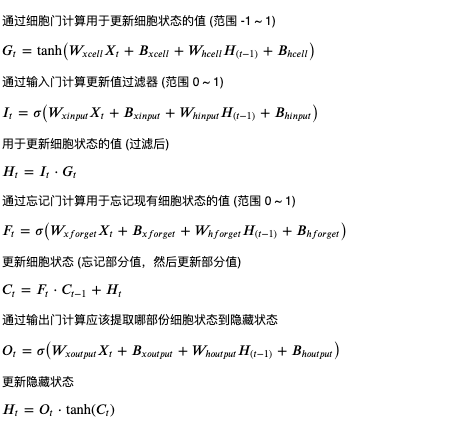(看不清请在新标签单独打开图片，或者另存为到本地以后查看)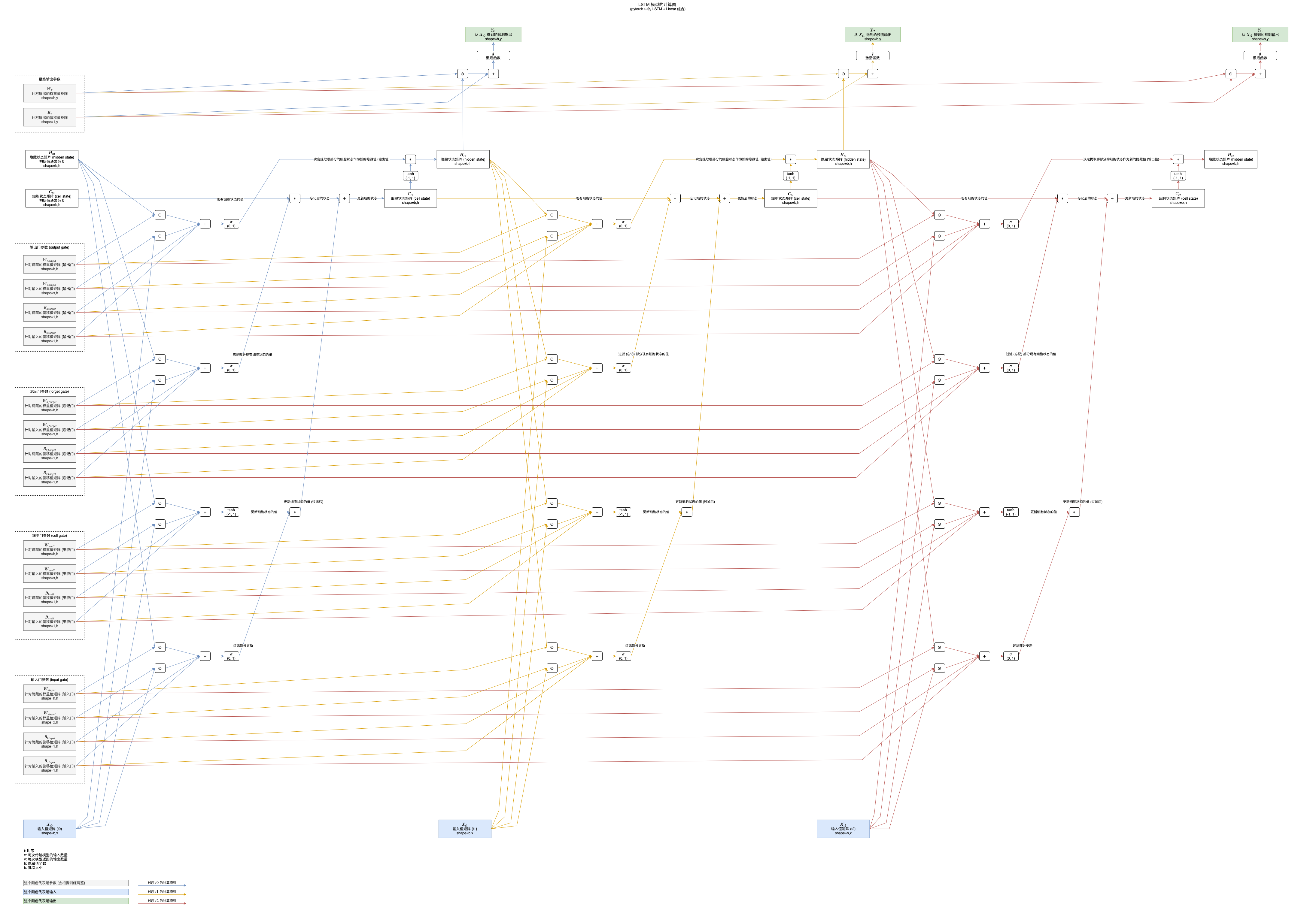LSTM 模型的细胞状态在叠加的过程中只会使用乘法和加法，所以可以避免梯度消失问题，并且输入门可以决定输入是否重要，如果不重要则减少对细胞状态的影响 (返回接近 0 的值)，忘记门可以在遇到某些输入的时候忘记细胞状态中记录的部分值，输出门可以根据输入决定如何提取细胞状态到隐藏值 (输出)，这些门让 LSTM 可以学习更长的数据，并且可以发挥更强的判断能力，但同时也让 LSTM 的计算量变大，需要使用更长的时间才能训练成功。

## 简化版长短记忆模型 - GRU

GRU (Gated Recurrent Unit) 是简化版的长短记忆模型，去掉了细胞状态并且只使用三个门，新增门负责计算更新隐藏状态的值，重置门负责过滤部分更新隐藏状态的值 (针对根据之前隐藏值计算的部分)，更新门负责计算更新比例，具体的计算公式如下：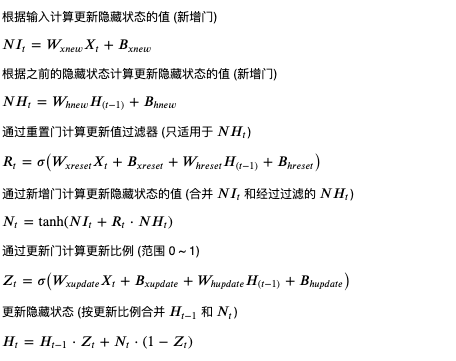(看不清请在新标签单独打开图片，或者另存为到本地以后查看)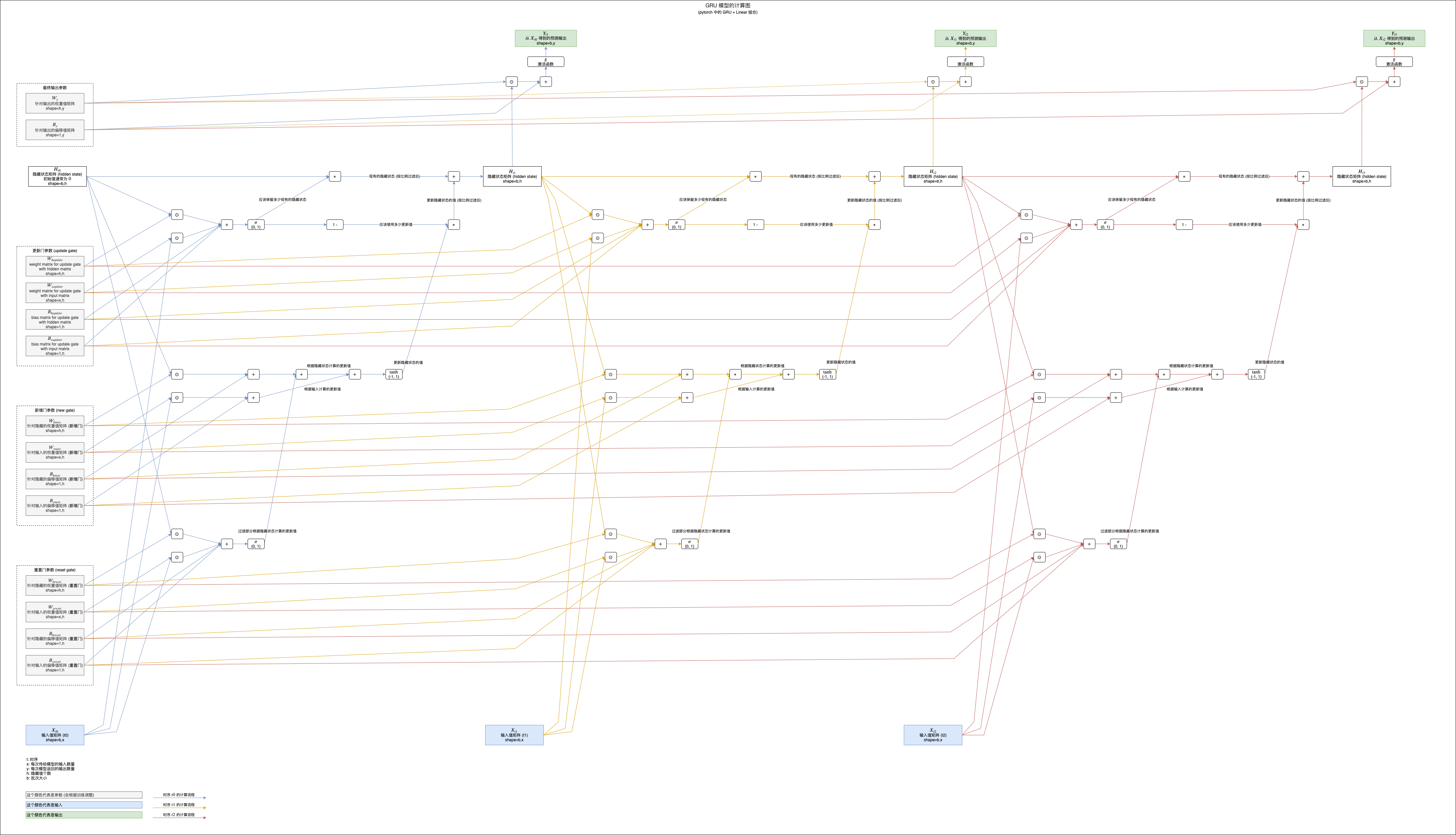## 在 pytorch 中使用 LSTM 和 GRU

pytorch 帮我们封装了 LSTM 和 GRU 模型，基本上我们只需要替换代码中的 RNNLSTMGRU 即可，示例代码如下：

>>> rnn_model = torch.nn.RNN(input_size = 1, hidden_size = 8, num_layers = 1, batch_first = True)

>>> rnn_model = torch.nn.LSTM(input_size = 1, hidden_size = 8, num_layers = 1, batch_first = True)

>>> rnn_model = torch.nn.GRU(input_size = 1, hidden_size = 8, num_layers = 1, batch_first = True)


## 写在最后

posted on 2020-05-28 17:26  q303248153  阅读(1213)  评论(3编辑  收藏